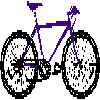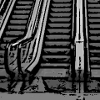#### You may also likeFour vehicles travelled on a road. What can you deduce from the times that they met?### There and Back

Brian swims at twice the speed that a river is flowing, downstream from one moored boat to another and back again, taking 12 minutes altogether. How long would it have taken him in still water?### Escalator

At Holborn underground station there is a very long escalator. Two people are in a hurry and so climb the escalator as it is moving upwards, thus adding their speed to that of the moving steps. ... How many steps are there on the escalator?

# Speeding Boats

##### Age 14 to 16Challenge Level

Uzair from Wilson's School, and Katherine from St Joseph's School both correctly said that at the same speed, boats would always meet in the middle, and if one was going twice as fast as the other they would meet $\frac{2}{3}$ of the way across.

Philip from Wilson's School continued this line of thinking to explain in general where the boats will meet:
When both boats start at opposite ends and travel at constant speed, their first meeting will be halfway down the lake and every meeting thereafter will occur halfway down the lake.

If the ratio of the boats is $2:1$, then boat A is travelling at twice the speed as boat B. They would first meet $\frac{2}{3}$ of the way down the lake. Their second meeting would be at the end of the lake, after boat A has gone two lengths and boat B one.

If the ratio is $3:1$, with boat A going three times as fast as boat B, they would first meet $\frac{3}{4}$ of the way down the lake. Their next meeting would be $\frac{1}{4}$ of the way down the lake, after boat A has gone 2.25 lengths and boat B 0.75 lengths.

If the ratio is $4:1$, with boat A going four times as fast as boat B, they would first meet $\frac{4}{5}$ of the way down the lake.

There is a consistent pattern here: say the amount of times boat A is going faster than boat B is $n$. They would then meet $\frac{n}{n+1}$ of the way down the lake.

Robert clearly explained that boat B must travel further than boat A:

No matter what speed the boats are travelling at, the lake cannot be $600$m or any smaller than that distance. If the lake was smaller than $600$m, the two boats wouldn'??t be able to get $600$m from side A. If the lake was $600$m long, that suggests that boat B would not be moving, however this would be impossible as boat B needs to get $400$m from his starting point for the second crossing.

Boat B must travel further than Boat A. For example, if the lake was $1000$m long, Boat A travels $600$m for the first crossing, and Boat B travels $400$m for the first crossing. Then Boat A has to travel $400$m to the end of the of the lake, and Boat B $600$m to the end of the lake, at this point both boats have travelled $1000$m. Now Boat A has to travel $400$m to the second crossing point $400$m from side B, and Boat B has to travel $600$m to the second crossing point.
So altogether, Boat A has travelled $600 + 400 + 400 = 1400$m and Boat B has travelled $400 + 600 + 600 = 1600$m.

If the lake is $1500$m long, Boat A travels $600$m to the first crossing point, and Boat B travels $900$m to the first crossing point. Next, Boat A has to travel $900$m to the other side of the lake, and Boat B only has to travel $600$m to the other side of the lake. At this point both boats have travelled $1500$m. For the final leg Boat A has to travel $400$m to the second crossing point, and Boat B has to travel $1100$m to the second crossing point.
So altogether, Boat A has travelled $600 + 900 + 400 = 1900$m and Boat B has travelled $900 + 600 + 1100 = 2600$m.

In general, Boat A's distance travelled is Length of lake + $400$m
Boat B's distance travelled is Length of lake $+$ (Length of lake + $400$m).

Extending Robert's ideas:
We know boat B travels further than boat A, so the lake must be more than $1200$m long, as Boat A has travelled $600$m when they meet for the first time.

Let's say the first meeting happens after $1$ unit of time. Then the second meeting is more than $1$ unit of time later as A has to complete the length and turn the corner.

After $3$ units of time, A has travelled $1800$m. If this was the second meeting point, A has travelled Length of lake + $400$m, so the lake must be $1400$m. This would suggest that Boat B travels $800$m in $1$ unit of time, so travels $2400$m in three units of time.

The second meeting point is when Boat B has travelled $1400 + (1400 - 400) = 2400$m, so the solution is that the lake is $1400$m long and Boat A travels at $\frac{3}{4}$ of the speed of Boat B.